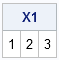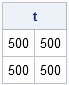I recently blogged about how to eliminate a macro loop in favor of using SAS/IML language statements. The purpose of the program was to extract N 3x3 matrices from a big 3Nx3 matrix. The main portion of my PROC IML program looked something like this:

```proc iml; ... do i=0 to N-1; rows = (3*i+1):(3*i+3); /** find certain rows **/ s = X[rows,]; /** extract those rows **/ /** do something with s **/ end;```

A reader correctly pointed out that my version of the program does not enable the programmer to access multiple matrices simultaneously. For example, after looping through all the rows of the data, the programmer cannot compute the sum of several of the 3x3 matrices. In my version, each 3x3 matrix is overwritten by the next.

However, you can address this issue by using the VALSET and VALUE functions. Never heard of them? Not very many people have, but they provide a mechanism to indirectly assign a value to a matrix name, or to indirectly get the value of a matrix name.

### Indirect Assignment of Values to Matrices

A simple example illustrates the usage of the VALSET function. Suppose you want to assign values to a matrix named x1. The usual way is to write x1={1 2 3}, but you can use the following statements to achieve the same result:

```/** indirect assignment: create a matrix name and assign a value to it **/ VarName = "x1"; call valset(VarName, {1 2 3}); print x1;```So what use is that? Well, 99% of the time it's of no use at all! However, this kind of "indirect assignment" is useful in the situation where you need to assign many matrices, and the assignment is most easily done in a loop.

For example, suppose you want to assign the matrix x1 to be a 2x2 matrix that consists entirely of 1s, the matrix x2 to be a 2x2 matrix that consists entirely of 2s, and so forth, up to x1000. Furthermore, suppose that you want all of these matrices available for future computations.

You have three options. You can write 1,000 literal assignment statements, you can write a macro %DO loop, or you can use the VALSET function. As I described in a previous blog, the following statements create the matrix names (x1,...,x1000) by using the string concatenation operator (+) in conjunction with the CHAR function (which converts a number into a character string) and the STRIP function. The VALSET function then assigns a value to each matrix:

```do i = 1 to 1000; VarName = "x" + strip(char(i,4)); /** x1, x2,... **/ v = repeat(i, 2, 2); /** create 2x2 matrix **/ call valset(VarName, v); end;```

You can use the SHOW NAMES statement to convince yourself that the matrices x1,...,x1000 are assigned. You can also compute with any or all of these matrices:

```t = x1 + x13 + x7 + x29 + x450; print t;```### Indirect Retrieval of Values from Matrices

The VALSET function sets a matrix to a value. The VALUE function retrieves the value. For example, the following statement gets the value in the x1 matrix:

`v = value("x1");`

This syntax is rarely necessary, but it can be useful to retrieve values within a loop. For example, to compute the sum x1 + x13 + x7 + x29 + x450, you can define a vector that contains the suffixes, and then create the name of each matrix and use the VALUE function to retrieve its value, as shown in the following statements:

```/** indirect reference: create a matrix name and retrieve its value **/ k = {1 13 7 29 450}; t = j(2, 2, 0); /** initialize to zero matrix **/ do i = 1 to ncol(k); VarName = "x" + strip(char(k[i],4)); /** x1, x13,... **/ t = t + value(VarName); end;```

In conclusion, the VALSET and VALUE functions in SAS/IML are little-known functions that can be used to assign and retrieve values from matrices. They can sometimes be used to replace the functionality of a %DO loop. They can also be used for other purposes, such as to produce the functionality of multi-dimensional arrays in PROC IML.

ShareDistinguished Researcher in Computational Statistics

Rick Wicklin, PhD, is a distinguished researcher in computational statistics at SAS and is a principal developer of SAS/IML software. His areas of expertise include computational statistics, simulation, statistical graphics, and modern methods in statistical data analysis. Rick is author of the books Statistical Programming with SAS/IML Software and Simulating Data with SAS.

1.One thing that would really be nice to see in IML is support for more data types than just matrices and vectors. Multi-dimensional arrays would be nice, and are a perfect fit for the kind of situation described here. A 3-dimensional array would let you organise the data in a way that reflects how it'll be used, without having to manage multiple variables or compute offsets into one big matrix.

2.Rick Wicklin on

I couldn't agree more.

3.Thanks Rick I just used VALSET in a programme, once again your blog was the way I learned it. Further question: having used CALL VALSET I want to attribute formats and column/row names to the resulting matrix. Usually I would use MATTRIB but I can't figure out how to point to the indirectly created matrix name in the MATTRIB statement (without literally writing it which defeats the purpose - I need to be able to automatically insert the new matrix name and attribute to it).

•You might want to examine CALL EXECUTE. Alternately, CALL SYMPUT (or SYMPUTX) would probably work.

4.One extra note on this topic. In a usual DATA step you can use the index variable in a DO loop to create new variable names, for instance if i is the index (say do i = 1 to 10 by 1) then "x_i = " will create x_1, x_2 etc. Once of the reasons for VALSET is that IML cannot assign matrix names in this way, which seems odd. My previous post would not be an issue if IML could.

•In a matrix language, variables named x1, x2, x3... are rarely needed because you can pack the contents of those variables into rows of a big matrix X.
Instead of a variable named x3, I typically store what I need in X[3, ].

•PS. I do not understand your DATA step code. Can you give a full example of what you mean?

5.Thanks for the advice will check CALL EXECUTE etc. Regarding your DATA step clarification request: just realized I was wrong. I have created multiple variables using an index number in a DO loop within a data step before, but I can't for the moment recall the syntax I used and my tests are proving wrong. Will try to recall: in the meantime, VALSET is a great solution.

Warm regards

6.Hi Rick having an issue with CALL VALSET sorry to bug you but hoping you can de-bug me :-)

Testing a do-loop, using one iteration to test it. I've created the scalar of a character name and a matrix of ones and zeros as follows, then trying to name the matrix using call valset:

STAR_NAMES = "LESS_" + STRIP(CHAR(PVALUES[,1]));
STAR_VALUES = STRIP(CHAR((P_VALUES <= PVALUES[,1])#NOT_MISS));
CALL VALSET(STAR_NAMES, STAR_VALUES);

Prints and tests of the two matrices confirm that they work, however, the CALL VALSET produces an "ERROR: (execution) Invalid argument to function".

It seems it may be the lengths of the respective matrices: if I simply change the content of the STAR_NAMES matrix to "A" it works. Any ideas welcome.

•Never mind figured it out: it was the "." in the name variable :-)